AP State Syllabus AP Board 9th Class Physical Science Important Questions Chapter 1 Motion.

## AP State Syllabus 9th Class Physical Science Important Questions 1st Lesson Motion

### 9th Class Physical Science 1st Lesson Motion 1 Mark Important Questions and Answers

Question 1.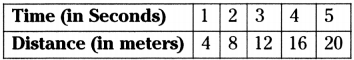From the above data, what can you say about the motion of the object?
The object is in the uniform motion and its speed is constant.

Question 2.
Frame any one question to understand Newton’s third law of motion.
How does rocket engine work?

### 9th Class Physical Science 1st Lesson Motion 2 Marks Important Questions and Answers

Question 1.
Distinguish between speed and velocity. jawjMB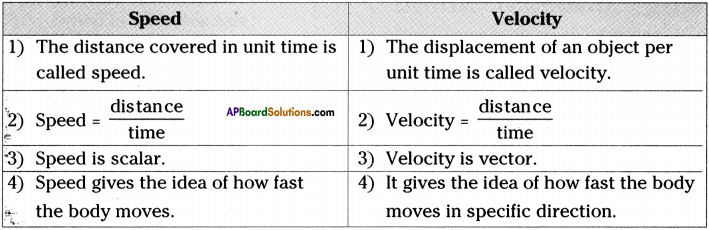Question 2.
What happens to the speed and the direction of motion of a ball rolling down an inclined plane?

1. Speed of the rolling ball changes.
2. But the direction of motion remains constant.

Question 3.
A motor cyclist drive from A to B with uniform speed of 30 km/hour and returns back with a speed of 20 km/hour. Find the average speed.
Speed of the motor cyclist from A to B = 30 km/hr.
Speed of the motor cyclist from B to A = 20 km/hr.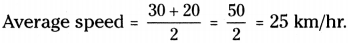Question 4.
A train of length 100 m. is moving with a constant speed of 10 m/s. Calculate the- time taken by the train to cross the electric pole.
Length of the train = 100 m; Speed of the train = 10 m/s
Time taken to cross the elctrical pole (t) = s/v = $$\frac{100}{10}$$ =10 sec.
[∵ Distance travelled while crossing an electrical pole = Length of the train]Question 5.
Calculate the average speed of “Ussahi Bolt” who sprints 100m in 9.81 sec. during 2016 Rio Olympics to win Gold medal.
Distance covered by Ussain Bolt = 100 m
time = 9.81s.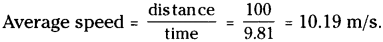Question 6.
Explain the terms in the formula v = u + at.
v = u + at
u = initial velocity
v = final velocity
a = acceleration
t = time

### 9th Class Physical Science 1st Lesson Motion Important Questions and Answers

9th Class Physical Science 1st Lesson Motion 1 Mark Important Questions and Answers

Question 1.
Define uniform acceleration.
Acceleration is uniform when in equal intervals of time, equal changes in velocity occur.Question 2.
Write an equation of motion to find the distance travelled when initial velocity, time, and acceleration are given.
s = ut + $$\frac{1}{2}$$ at²

Question 3.
Draw a displacement vector from Visakhapatnam to Hyderabad to the following diagram.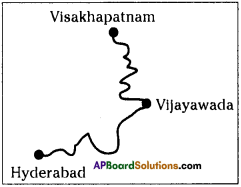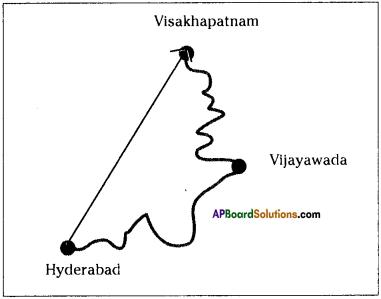Question 4.
Difine acceleration.
The rate of change of velocity in an object is known as its acceleration.

Question 5.
What is the key difference between distance and displacement?
Distance is the length of the path traversed by an object in a given time interval and displacement is the shortest distance covered by the object in a specific direction.Question 6.
The distance travelled by a particle in time’t’ is given by s = (2.5 m/s²) t². Find the average speed of the particle during the time ‘O’ to ‘5’ sec.
The distance travelled by the particle from 0 to 5 sec is s = 2.5 m/s² × 5² = 62.5 m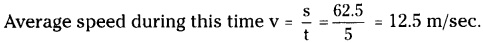Question 7.
A table clock has its minutes hand 4 cm long. Find the average velocity of the tip of the minute hand between 6.00 AM to 6.30 AM.
The minute hand of a clock comes into a straight line from 6.00 AM to 6.30 AM.
Hence the displacement of the minute hand S = 2 x 4 = 8 cm
Time = 30 minutes = 1800 sec.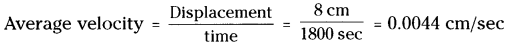Question 8.
A ball is thrown up with an initial speed of 4m/sec. Find the maximum height reached by the body.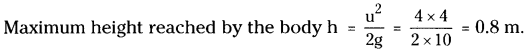Question 9.
The adjacent distance – time graph indicates
A) A particle travels constantly along X-axis.
B) Particle is at rest.
C) The velocity of the particle increases up to a time t0 and then remains constant.
D) The particle travels up to time t(( with constant velocity and then stops.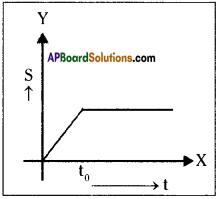C

Question 10.
A man used his car. The initial and final odometer readings are 4849 and 5549 respectively. The journey time is 70h. What is average speed of the journey?
Distance covered = 5549 – 4849 = 700 km. ; Time = 70 h.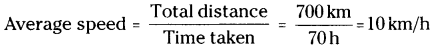9th Class Physical Science 1st Lesson Motion 2 Marks Important Questions and Answers

Question 1.
An object is moving in a circular path of radius 7 m. What is the distance and displacement of an object after one revolution ?
22
Distance covered by object = 2πr = 2 × $$\frac{22}{7}$$ × 7 = 44 m
The object come back to original position displacement of the object is 0.

Question 2.
An object reaches other end of a diameter in a circular path of radius 7 m in 7s. Find speed and velocity of the object?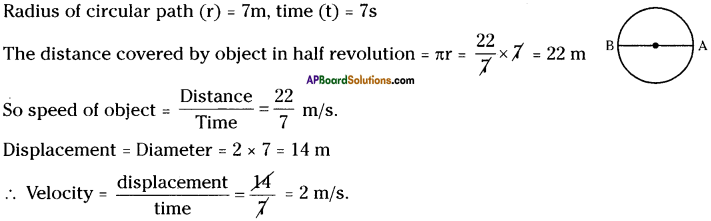Question 3.
A person moves 3 km towards east and turned toward north and travelled a distance of 4 km. Find total distance and total displacement.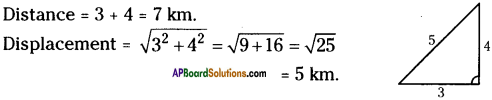Question 4.
An object completes 1/4th revolution of a circular path of radius r. Then find the ratio of distance and displacement.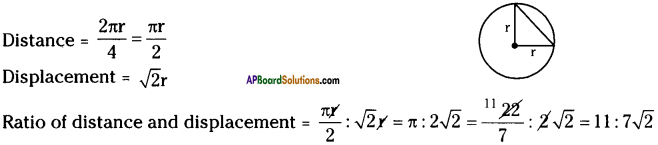Question 5.
a) What is a vector? Give example.
b) What is a scalar? Give example.
a) Vector :
The physical quantity which is specified with magnitude and direction is called a vector.
Eg : Displacement, velocity are vectors.

b) Scalar :
The physical quantity which does not require any direction for its specification is called ‘scalar’.
Eg : Distance, time are scalars.Question 6.
How does a vector represents? Explain.

1. A vector can be represented as a directed line segment.
2. It’s length indicates magnitude and arrow indicates it’s direction.
3. Vector is represented by an arrow as shown in the figure.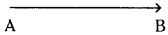Here point ‘A’ is called tail and point ‘B’ is called head.

Question 7.
Bhavan did not recognise the difference between 40 meters displacement and distance. Then Anitha ex- plained the difference by asking some questions to Bhavan. What would be those questions?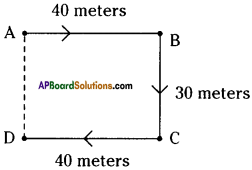Note : She used this diagram.
By using the diagram Anitha asked the following questions :

1. What is the distance between A and D?
2. Which is the shortest distance from A to D?
3. What is the direction of an object if it travelled in the route A → B → C → D? Can you determine its direction?
4. On which direction the distance is short from A to D? Measure it.
5. Which path has specified direction?
Either A → B → C → D or A → D?
6. Which path has specified magnitude?
Either A → B → C → DorA → Dor Both?
7. Which is the shortest, distance among the two paths?
8. If the displacement has both magnitude and specified direction, which path is considered as a displacement?
9. What is the difference between distance and displacement?

Question 8.
What happens to the direction of velocity and acceleration when the
i) speed of an object increases?
ii) speed of an object decreases?
i) If the speed of an object increases, the direction of velocity and acceleration are one and the same.
ii) If the speed of an object decreases, the direction of velocity and acceleration are in opposite directions. Here, at a certain instant the speed becomes zero.Question 9.
Draw a displacement vector and a velocity vector to the given path of motion of a body.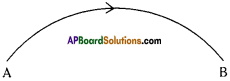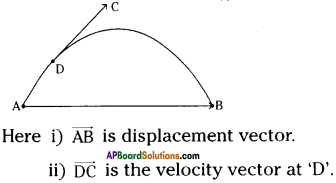Question 10.
Find the acceleration of a bus if its speed increases from 0 m/s to – 600 m/s in 1 minute?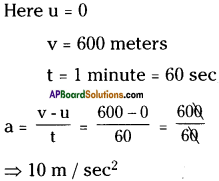The acceleration of the bus is 10 m/sec².

9th Class Physical Science 1st Lesson Motion 4 Marks Important Questions and Answers

Question 1.
Distinguish between distance and displacement.

 Distance Displacement 1. Distance is the length of the path travelled by an object in a given time interval. 1. Displacement is the shortest distance covered by the object in a specified direction. 2. SI unit of distance is ‘meter’. 2. SI unit of displacement is ‘meter’. 3. Distance is scalar. 3. Displacement is vector. 4. Distance will not be zero even if it reaches the initial position after its journey. 4. Displacement will become zero, if it reaches its initial position after its journey.

Question 2.
Explain the terms uniform and non-uniform motions.
Uniform motion :

1. If a body covers equal distances in equal intervals of time, assuming the direction of motion is constant, then the motion is said to be uniform motion.
2. The motion of a body is said to be uniform when its velocity is constant.
3. The s -1 graph for a uniform motion is a straight line.
4. Ex : Motion of hands in a clock.

Non-uniform motion :

1. If a body covers unequal distances in equal intervals of time, assuming the direction of motion is constant, then the motion is said to be non-uniform motion.
2. A body is said to be in non-uniform motion if its velocity changes with time.
3. The s -1 graph for non-uniform motion is not a straight line.
4. Ex : Motion of a car/bus between two stations on a road.Question 3.
Derive equations of uniform accelerated motion.
1) Let ‘u’ be the velocity at the time t = 0 and V be the velocity at the time’t’ and let ‘s’ be the displacement covered by the body during time’t’ as shown in figure.
2) From the definition of uniform acceleration,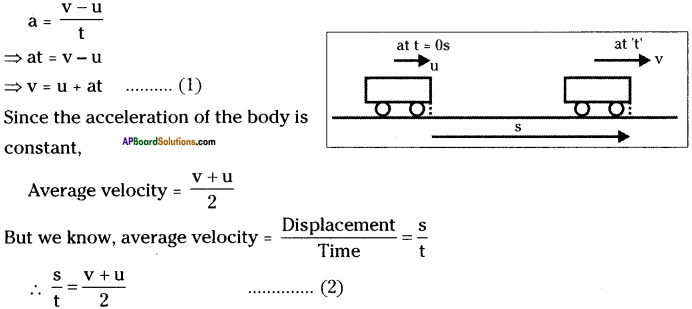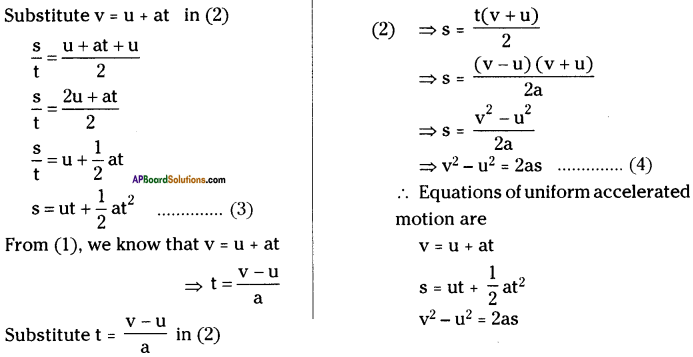Question 4.
Describe the graphical method to calculate the instantaneous speed at a given point.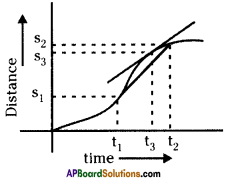1. Consider a car moving along a straight road with varying speed.
2. Take time elapsed on X – axis and distance cov¬ered on Y – axis and plot a graph for its motion at regular intervals of time.
3. A general case of motion with varying speed is shown in the figure.
4. The average speed during the time interval from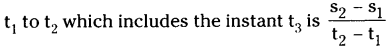5. Then we calculate average speed for a very short time interval encompassing the time at an instant t3 which is so short interval, that value of average speed would not change materially if it was made even shorter.
6. The instantaneous speed is represented by the slope of the curve at a given instant of time.
7. The slope can be found by drawing a tangent to the curve at that point.
8. The slope of the curve gives speed of the car at that instant.

Question 5.
What is difference between speed and velocity?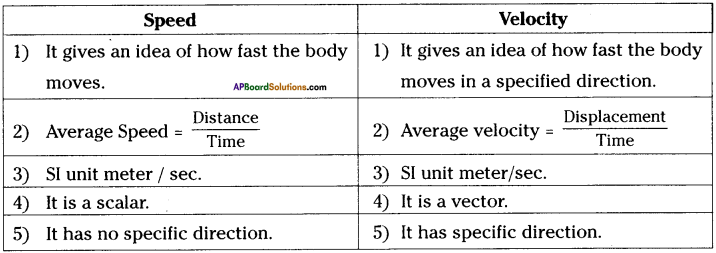Question 6.
Derive s = ut + $$\frac{1}{2}$$ at²
Derive one equation for displacement of a body which is in the uniform acceleration.
1) Let u be the initial velocity,
v be the velocity at time ‘t’.
a be the acceleration,
s be the displacement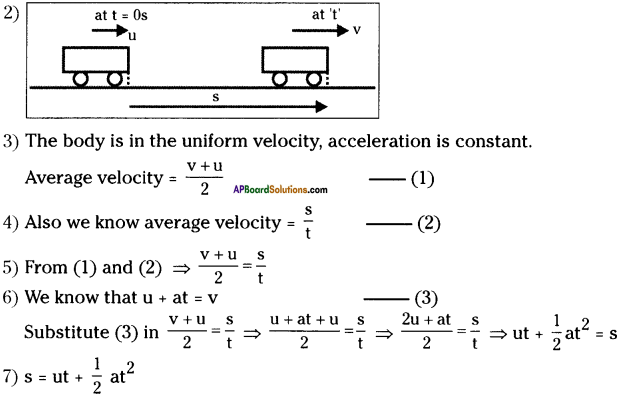Question 7.
A car travelled from A to E station in 3 minutes. Viewing path given below in the figure. find
i) distance
ii) displacement
iii) speed
iv) velocity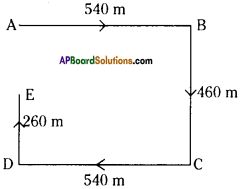i) Distance = AB + BC + CD + DE = 540 + 460 + 540 + 260 = 1800 m

ii) Displacement = Distance between A and E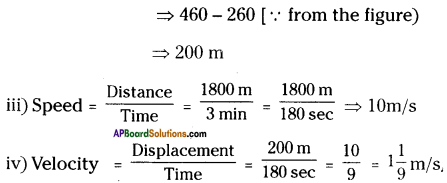Question 8.
Give an example to each situation in daily life.
i) Speed changes when direction remains constant.
ii) Direction of motion changes when speed remains constant.
iii) Speed and direction simultaneously change.